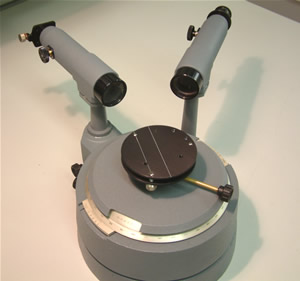# diffraction grating simulation

We used the grating equation$n \lambda = d \sin \theta$

today to predict the location of bright fringes produced by a diffraction grating.  Remember that d in this equation is the distance between adjacent lines in the grating and not the number of lines per metre/millimetre/inch. We’ll look at applications of this equation a little more this week, e.g. using a spectrometer to measure the angle so we can calculate the wavelength of the light used.###### spectrometer

In the meantime, get some practice at using the grating equation with the simulation site shown below.  You can select how many lines you would like per millimeter of grating and alter the wavelength.  Try calculating the angle for the first or second order spots and then use the simulated protractor to see if you are correct.  Click on the image below to get started.### 0 thoughts on “diffraction grating simulation”

This site uses Akismet to reduce spam. Learn how your comment data is processed.